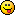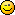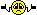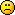Math Is Fun Forum

Discussion about math, puzzles, games and fun.   Useful symbols: ÷ × ½ √ ∞ ≠ ≤ ≥ ≈ ⇒ ± ∈ Δ θ ∴ ∑ ∫  π  -¹ ² ³ °

You are not logged in.

#1 Help Me ! » Law of sines and Trig » 2018-11-05 18:07:04

careless25
Replies: 1

I am trying to help my cousin in High School solve the following problem:
Solve for x

Now I can solve all the sides and angles on the top triangle but I can't find a way to solve anything else on the bottom trianlge.

I am guessing that we need to either get some congruent triangles or draw triangles in here to get the third piece of information thats missing from the bottom triangle. I just can't figure out where or how to start on that.

Any help would be appreciated

#2 Re: Help Me ! » Combinations with repetitions problem » 2015-01-18 09:04:00

Yes those are also different.

#3 Re: Help Me ! » Combinations with repetitions problem » 2015-01-18 08:17:37

anon: Yes both flavors xi and yi are the same.

Elaina: No those two are not the same, they differ since both the yi's and si's are different. No both flavours can be same as long as the size is different.

#4 Re: Help Me ! » Combinations with repetitions problem » 2015-01-18 05:22:12

One example of 12 coffees would be:

1. (x1, y1, s1)
2. (x1, y1, s2)
3. (x1, y1, s3)
4. (x2, y1, s1)
5. (x2, y1, s2)
6. (x2, y1, s3)
7. (x3, y1, s1)
8. (x3, y1, s2)
9. (x3, y1, s3)
10. (x4, y1, s1)
11. (x4, y1, s2)
12. (x4, y1, s3)

4 to 6 are equivalent to (x1, y2, s1), (x1, y2, s2), and (x1, y2, s3). So do not double count these.
Same goes for 7-9 and 10-12.

#5 Help Me ! » Combinations with repetitions problem » 2015-01-18 04:40:20

careless25
Replies: 9

I am stuck at a combination problem. I do not know how to logically organize the given items.

The question is:

A coffee shop sells 30 different flavours of coffee and it offers 3 different sizes. In how many ways can we order a dozen two-flavoured coffees if any two of them in one order must differ at least by the flavour or by the size?

I tried to do the following:

X = flavour 1 => 30 flavours

Y = flavour 2 => 30 flavours

S = size => 3 sizes

Now I have to find a way to first select 3 items from the sets above and then 11 more distinct ways to select those 3 items.

So the first one I have the choice of all 30 flavours for both X and Y and any size. So I can select the first one 30*30*3 ways.

Next one I can select either with a different flavour for X or Y or a different size. So the two ways are: 30*29*3 or 30*30*2

If I follow this logic through it gets into too many different cases and its hard to keep track of. Is there a better way to do it?

#6 Re: Help Me ! » Correlation Coefficient » 2014-08-08 05:43:33

I must have messed up some of my signs somewhere.

Thanks Bob!

EDIT:

Actually how is V[X1+X2+X2+X3] = 6?? shouldn't it be 4 as V[X1 + 2*X2 + X3] = V[X1] + 2*V[X2] + V[X3] = 1+2+1?

#7 Re: Help Me ! » Correlation Coefficient » 2014-08-08 02:00:01

Hi Bob Bundy,

I am asking for the Correlation coefficient of X1 + X2 and X2+X3. Not X1+X2 and X3+X4.

I agree with your answer if it was that.

#8 Help Me ! » Correlation Coefficient » 2014-08-07 04:49:47

careless25
Replies: 7

This was a question on my exam and I am not sure I did it correctly. Can someone show me the steps to derive it?

Given X1, X2, X3, X4 are random uncorrelated variables with variance of 1 and mean of 0. Find the correlation coefficient for (X1 + X2 and X2 + X3).

I got the answer as -1/2. is this correct?

#9 Re: Help Me ! » Probability Random Variable with Central Limit Theorem » 2014-08-02 17:44:21

Hi bobbym,

Var(X) = E(x^2) - E(x)^2

and f(x) = 1 as this is uniform distribution from -1/2 to 1/2.

so:

Using Central Limit Theorem:

is the square root of the variance calculated above.
is the standard distribution.

Therefore P(-2 <= x <= 2) = 0.512

#10 Help Me ! » Probability Random Variable with Central Limit Theorem » 2014-07-31 22:53:11

careless25
Replies: 2

At a bank, the interest is calculated on 100 accounts and rounded up or down
to nearest cent. If it is assumed that the round-off error is uniformly distributed between
(-1/2, 1/2) and
the round-off errors are independent, find the probability that the sum
of the error does not exceed 2 cents in magnitude.

I dont understand how to calculate the variance for just one account. the answer for variance for just one account is 1/12.

#11 Re: Euler Avenue » Which Prime below 1 million can be expressed as the sum of most primes » 2012-08-13 02:07:11

It should be the largest number which is the sum of the maximum number of distinct primes.

#12 Re: Help Me ! » Exponents and Scientific Notation » 2012-08-12 16:22:51

EDIT: Removed, to not confuse OP

#13 Re: Help Me ! » Adding and Subtracting Polynomials » 2012-08-12 15:58:22

Hey

The negative sign does not "belong" to x, think of it as -1 * x = -1x = - x.

Does that make sense?

#14 Re: Euler Avenue » Which Prime below 1 million can be expressed as the sum of most primes » 2012-08-11 10:07:22

Agnishom, I will post the answer here but I got it by chance, so I cannot tell you how to code it.

#15 Re: Help Me ! » Adding and Subtracting Polynomials » 2012-08-11 08:41:48

hi,

Almost correct! It is

#16 Re: Euler Avenue » Which Prime below 1 million can be expressed as the sum of most primes » 2012-08-11 08:40:14

Hi bobbym,

That is not a prime. 997661 = 7*359*397#17 Re: Euler Avenue » Which Prime below 1 million can be expressed as the sum of most primes » 2012-08-10 18:25:04

Btw i just solved it by chance.

Good luck...also i believe after prime 550, the sum come to more than a million, if you start from the 4th prime.

#18 Re: Euler Avenue » Which Prime below 1 million can be expressed as the sum of most primes » 2012-08-10 18:12:17

Hey,

I just did this in excel and I get 1074180, if i sum up from the 4th prime to the 565th prime.SO post your code and lets see where you went wrong.

Also just to make sure we are starting and ending at the same primes: 4th prime is 7 and 565th prime is 4099.

#19 Re: Help Me ! » 1=2 proof » 2012-08-08 14:37:52

Heres an infinite series "proof" that shows 0 = 1

0 = 0 + 0 + 0 + 0 + · · ·
= (1 − 1) + (1 − 1) + (1 − 1) + (1 − 1) + · · ·
= 1 − 1 + 1 − 1 + 1 − 1 + 1 − 1 + · · ·
= 1 + (−1 + 1) + (−1 + 1) + (−1 + 1) + · · ·
= 1 + 0 + 0 + 0 + · · ·
= 1

"Legend has it that, around 1703, in letters to contemporary mathematicians, an Italian monk by the name of Guido Grandi (often called Guido Ubaldus) presented this as proof of the existence of God, since it suggested that the universe could have been created out of nothing! What he actually meant isn't clear, but we do know that the brightest minds of the day were unsure how to explain what the problem was. Leibniz at least recognized that the problem was in the ____ line above;"

I left it blank intentionallyThis is copied from my Calculus prof notes, he showed is this "proof" in class.

#20 Re: Help Me ! » Integration » 2012-08-08 11:52:18

I have been looking at this and I realized that if we just square the actual integral and dont use the fomula given to us, we get to the correct answer.
This does not work:

but this does:

#21 Re: Help Me ! » Integration » 2012-08-08 10:34:05

Hi Bob bundy,

Can you verify my calculations below(just for my understanding):

now from here convert to polar co-ordinates
EDIT: I am not sure what the integration limits for theta would be...if it is 0 to pi/2, then this works out.

let u = r^2, du = 2rdr

since we squared the original equation, we square root the final answer so

This doesnt agree with what wolfram and wikipedia get. Any idea where I went wrong?

Thanks

#22 Re: Help Me ! » Adding and Subtracting Polynomials » 2012-08-08 07:45:05

The degree of the polynomial is the highest degree in the polynomial.
For example:

has 3 as the highest degree, so the degree of the polynomial is 3.

Adding and subtracting polynomials is just like adding and subtracting numbers. You collect the like terms(the terms which have the same degree) and add or subtract them together.

For example:

First you take care of the negative sign:

Then group the like terms together:

Follow these rules and try the questions you have posted again. Post your answers here, so we can see where you went wrong.

Cheers,
C25

PS Also look at the links bobbym posted

#23 Re: Help Me ! » Integration » 2012-08-08 06:16:10

Hahaha I could not have thought of that on the final exam. Lost 10% right there.

Thanks Bob

#24 Re: Help Me ! » Integration » 2012-08-08 04:56:48

Thanks bob bundy and bobbym.

Following that we were asked to use that to solve

and told to use polar coordinates.

I was confused on how to go from the above function to a function of x and y.

#25 Help Me ! » Integration » 2012-08-07 13:26:11

careless25
Replies: 8

Hi,

I just had a calc final and one question stumped me. But i would really love to know the answer. Can anyone help me solve this?
I have to show that: